GRE Subject Test: Physics : Atomic Spectra

Example Questions

Example Question #2 : Quantum Mechanics And Atomic Physics

What is the energy of the photon emitted when a Hydrogen atom makes a transition from the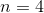to the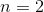atomic energy level?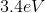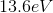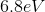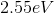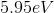Explanation: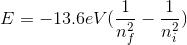, whererefers to the final and initial energy levels, respectively.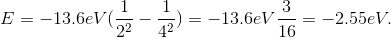However, since the question asked what is the energy of the photon, it is the absolute value of the energy calculated above because of conservation of energy. The photon carries away the energy lost by the atom.

Example Question #1 : Atomic Spectra

What color will be emitted in the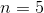totransition in the Hydrogen Balmer series?

Yellow

Red

Violet

Green

Blue

Violet

Explanation: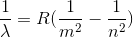.

Here,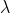is the wavelength,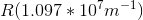is the Rydberg constant,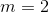for the Balmer series, andrefers to the upper atomic level (this isin this case). Now, we just need to plug everything in, and solve for wavelength.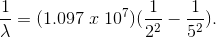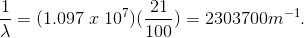By inverting,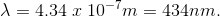This is in the violet regime of the visible spectrum.

All GRE Subject Test: Physics Resources